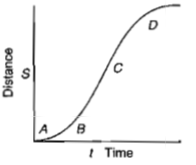The coordinate of an object is given as a function of time by $\mathrm{x}=7\mathrm{t}-3{\mathrm{t}}^{2}$, where x is in meters and t is in seconds. Its average velocity over the interval from t=0 to t=4 is:

(A)  5 m/s

(B)  -5 m/s

(C)  11 m/s

(D)  -11 m/s

Concept Questions :-

Average speed and average velocity

Difficulty Level:

A particle moves along a straight line and its position as function of time is given by $\mathrm{x}={\mathrm{t}}^{3}-3{\mathrm{t}}^{2}+3\mathrm{t}+3$ then particle

(A)  stops at t=1s and reverses its direction of motion

(B)  stops at t=1s and continues further without change of direction

(C)  stops at t=2s and reverses its direction of motion

(D)  stops at t=2s and continues further without change of direction

Concept Questions :-

Instantaneous speed and instantaneous velocity

Difficulty Level:

A particle moves in a straight line, according to the law , where x is its position in meters, t is in sec & a is some constant, then the velocity is zero at :

(A)

(B)

(C)

(D)  none

Concept Questions :-

Instantaneous speed and instantaneous velocity
High Yielding Test Series + Question Bank - NEET 2020

Difficulty Level:

A point moves in a straight line so that its displacement is x m at time t sec, given by ${\mathrm{x}}^{2}={\mathrm{t}}^{2}+1$. Its acceleration in $\mathrm{m}/{\mathrm{s}}^{2}$ at time 1 sec is:

(A)  $\frac{1}{\mathrm{x}}$

(B)  $\frac{1}{\mathrm{x}}-\frac{1}{{\mathrm{x}}^{3}}$

(C)  $\frac{1}{{\mathrm{x}}^{3}}$

(D)  $-\frac{{\mathrm{t}}^{2}}{{\mathrm{x}}^{3}}$

Concept Questions :-

Acceleration
High Yielding Test Series + Question Bank - NEET 2020

Difficulty Level:

The motion of a body is given by the equation, $\frac{dv}{dt}=4-2v$ where v is the speed in m/s and t in second. If the body was at rest at t=0, then find speed of body as a function of time.

1. $2\left(1-{e}^{-2t}\right)$

2. $1-{e}^{-2t}$

3. $2\left(1+{e}^{-2t}\right)$

4. $1-{e}^{-t}$

Concept Questions :-

Integration
High Yielding Test Series + Question Bank - NEET 2020

Difficulty Level:

A particle moves in a straight line with a constant acceleration. It changes its velocity from 10 to  while passing through  a distance 135 m in t second . The value of t is

(a) 10

(b) 1.8

(c) 12

(d) 9

Concept Questions :-

Uniformly accelerated motion
High Yielding Test Series + Question Bank - NEET 2020

Difficulty Level:

A particle shows distance-time curve as given in this figure. The maximum instantaneous velocity of the particle is around the point :1. B

2. C

3. D

4. A

Concept Questions :-

Graphs
High Yielding Test Series + Question Bank - NEET 2020

Difficulty Level:

The distance travelled by a particle starting from rest and moving with an acceleration $\frac{4}{3}m{s}^{-2},$ in the third second is

(a) 6m                             (b) 4m

(c) $\frac{10}{3}m$                         (d) $\frac{19}{3}m$

Concept Questions :-

Distance and displacement
High Yielding Test Series + Question Bank - NEET 2020

Difficulty Level:

A particle starts its motion from rest under the action of a constant force. If the distance covered in first 10 s is ${\mathrm{s}}_{1}$ and that covered in the first 20 s is ${\mathrm{s}}_{2},$ then

(a) ${\mathrm{s}}_{2}=2{\mathrm{s}}_{1}$                                     (b) ${\mathrm{s}}_{2}=3{\mathrm{s}}_{1}$

(c) ${\mathrm{s}}_{2}=4{\mathrm{s}}_{1}$                                      (d) ${\mathrm{s}}_{2}={\mathrm{s}}_{1}$

Concept Questions :-

Uniformly accelerated motion
High Yielding Test Series + Question Bank - NEET 2020

Difficulty Level:

A bus is moving with a speed of  on a straight road. A scooterist wishes to overtake the bus in . If the bus is at a distance of  from the scooterist, with what minimum speed should the scooterist chase the bus?

1.                                          2.

3.                                          4.

Concept Questions :-

Relative motion in 1-D